# Tableau Formatting Tip: How to Display Varying Decimal Places

• Author Experience
• Engineering
• Intermediate
• Tableau Desktop

This content is excerpted from my book, Innovative Tableau: 100 More Tips, Tutorials, and Strategies, published by O’Reilly Media Inc., 2020, ISBN: 978-1492075653. Get the book at Amazon.

I recently received a great question from a Twitter connection, and I figured I would share the solution here in case it helps anybody else. They were trying to display four decimal places when the measure value on the view was less than one, but only two decimal places when the measure value was greater than one. This was a brainteaser because, by default, Tableau limits you to one number format per measure.

I loved the concept, though, because I’m a big believer in maximizing the data-ink ratio, and the extra decimal places could be considered redundant data ink. My first instinct was to dynamically format the numbers with parameters, but this technique only works for controlling the prefix and suffix of each different number type. Instead, this post will show you two alternative approaches that allow you to control the prefix, suffix, and/or the number of decimal places when you are trying to display two (or even three!) number formats for the same measure in Tableau.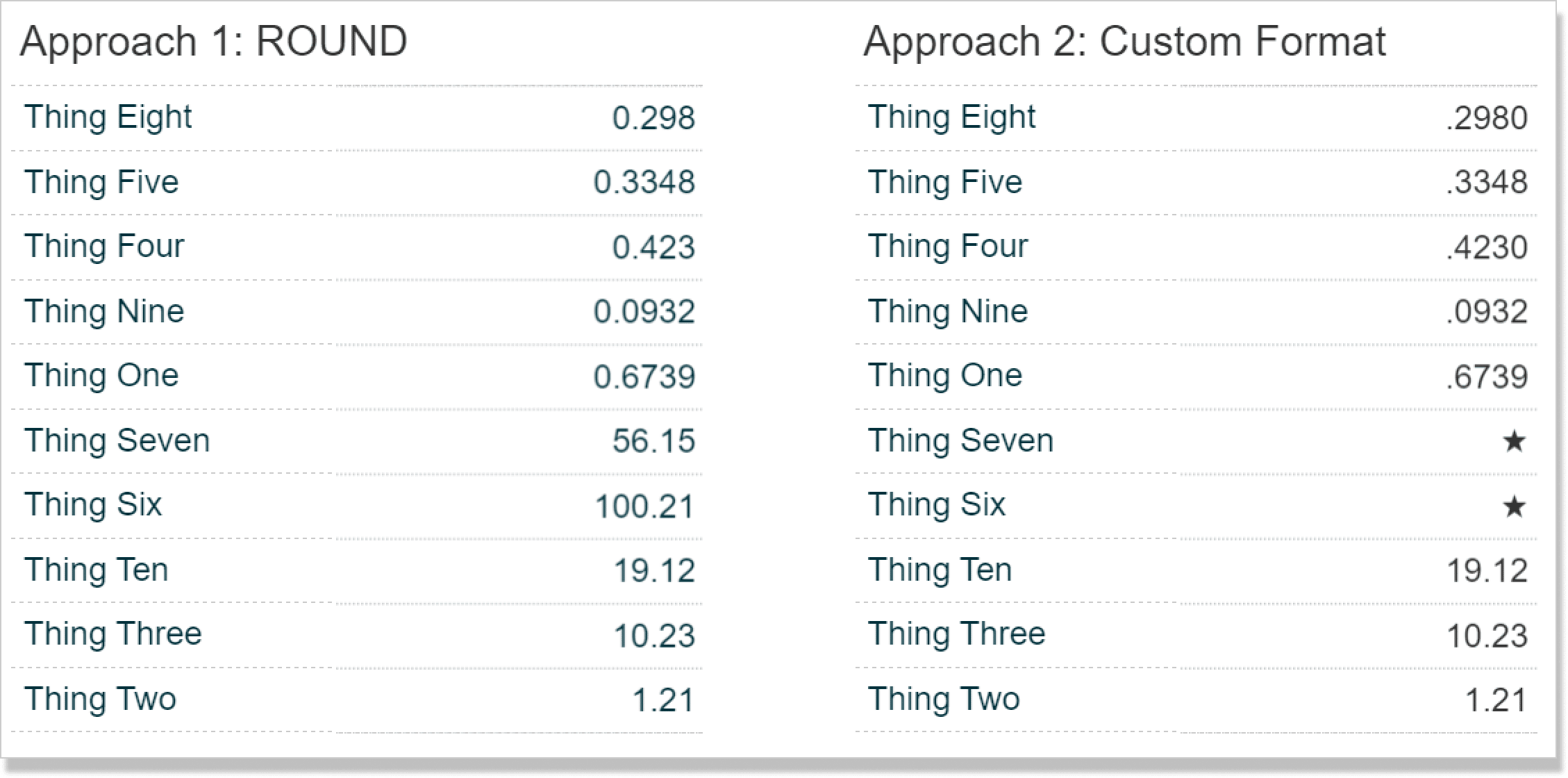## Using Tableau’s ROUND and IIF functions to display varying decimal places

This first approach was a tip I received from a friendly email subscriber. If your only objective is to round decimal places based on a measure’s value, you can use Tableau’s ROUND and IIF functions in a calculated field.

To illustrate the dynamic formatting, I’ve put together this dummy dataset with ‘ten things’. Note the measure values include varying levels of decimal places.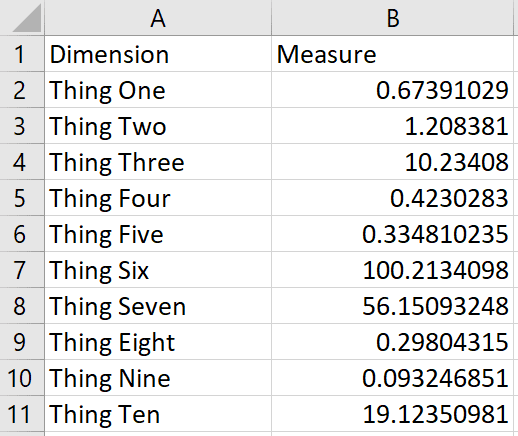If I were to make a table with these numbers in Tableau, the measure values would have “Automatic” formatting applied, and every number would display one decimal place.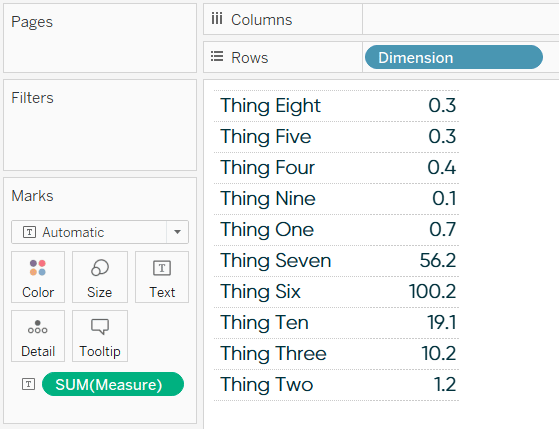I could either change the default formatting of the measure or change the format of the measure for this specific view to change the number of decimal places, but in both cases, all the numbers would share the same number of decimal places. If we are trying to display four decimal places for values that are less than one and two decimal places for numbers that are greater than one, we can use the following calculated field:

IIF([Measure]<1,ROUND([Measure],4),ROUND([Measure],2))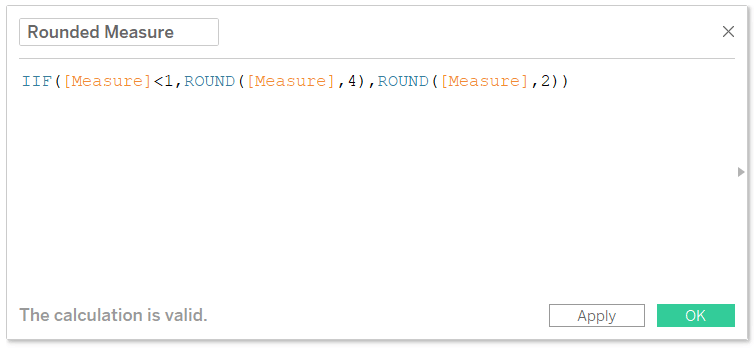This formula looks at the first statement, [Measure] < 1, and when that statement is true, Tableau will round the original measure to four decimal places. If that first statement is false, it rounds the measure to two decimal places. Now, replace the original measure from the dataset with the newly created calculated field.

### Receive More Free Tips & Tricks Like This Every Week

Let’s stay in touch:By default, you will still see “Automatic” formatting, and the measure values will be rounded to one decimal place. However, now when you change the number formatting to “Number (Standard)”, you will see the dynamic formatting appear. You can find this formatting by right-clicking on the measure that is currently on the Text Marks Card, choosing “Format”, and changing the format for the numbers on the pane (as opposed to axis). Values below one are rounded to four decimal places, and values above one are rounded to just two!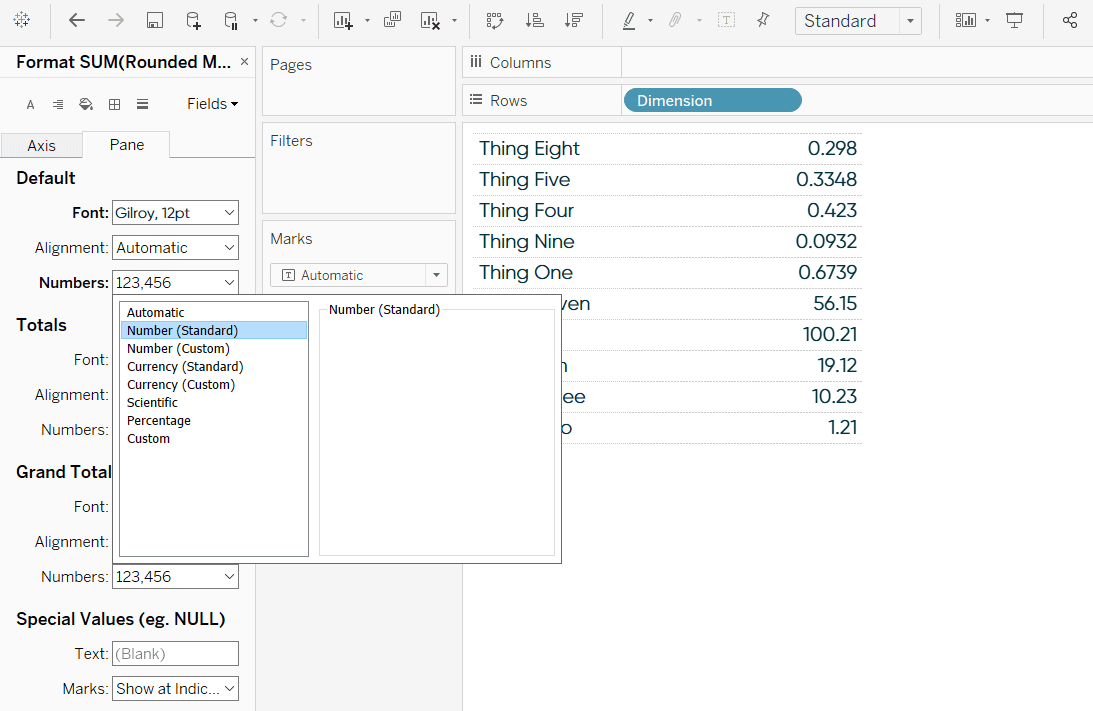The benefit of this approach is it works whether you’re formatting positive or negative values and elegantly solves the question at hand regarding decimal places. Read on for a solution that addresses broader applications.

## How to display two (or three) number formats for the same measure in Tableau

This next approach is more involved but unlocks more flexibility, including the ability to show a third variation as well as different number formats. I learned this trick from Tableau Zen Master Hall of Famer, Andy Kriebel, in his post One Metric; Two Number Formats. Andy used the technique to display different number types (i.e. percentages, currencies) when you are allowing users to select measures with parameters, but this also works for displaying different numbers of decimal places for the same measure.

First, create a calculated field that multiples whatever you want to have a unique formatting by negative one. For this scenario, we’ll say that two decimal places will be our default format, but for values less than one, we want to make an exception and display four decimal places. Therefore, the values less than one are the ones we multiply by negative one. The formula is:

IF SUM([Measure]) < 1 THEN -SUM([Measure])
ELSE SUM([Measure])
END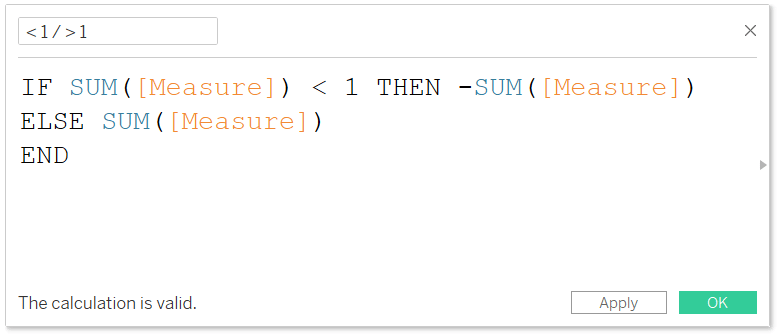Next, replace the original measure with the newly created calculated field that converts your exception to negative.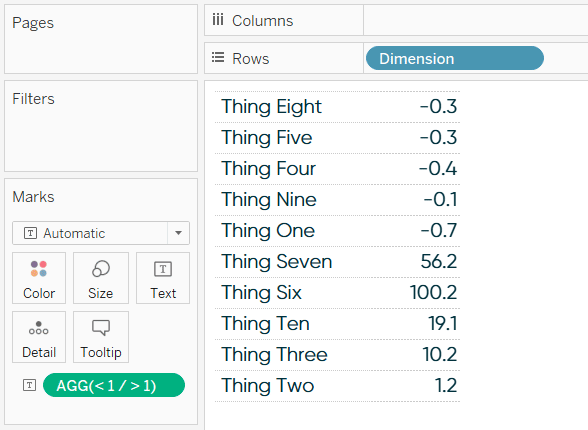Now that we have a mix of negative and positive values, we can apply a unique format to both types of values. This is accomplished by right-clicking on the measure on the view (currently on the Text Marks Card in my example), choosing “Format…”, and updating the format for the numbers on the pane. When you choose “Custom”, any format you type before a semicolon will be applied to positive numbers, and any format you type after a semicolon will be applied to negative numbers.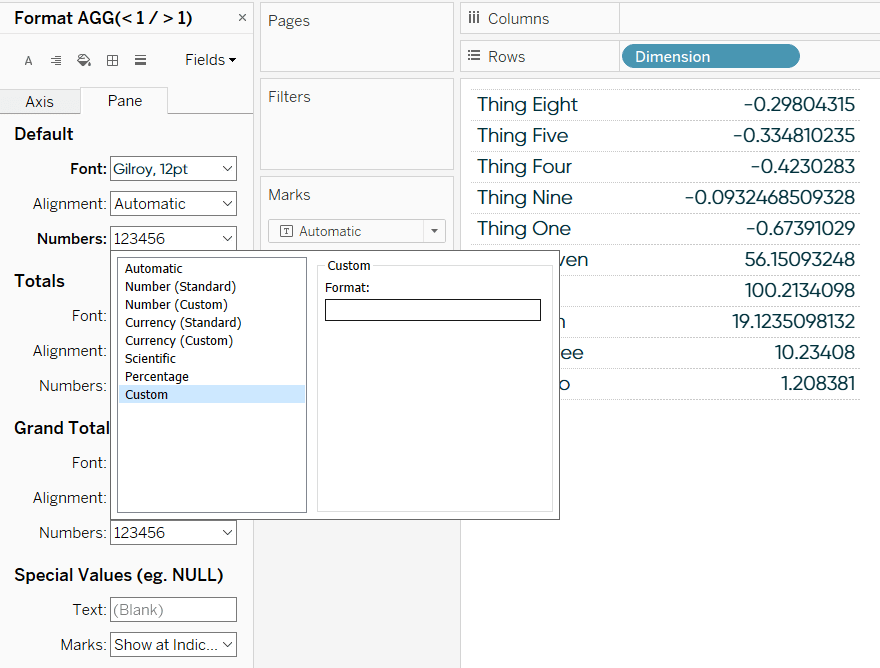If I type .00 before a semicolon in the custom text box, that number formatting will be applied to positive numbers. In my example, the positive numbers are the values above one. If I type .0000 after a semicolon, that format will be applied to negative numbers, or the values below one in my example.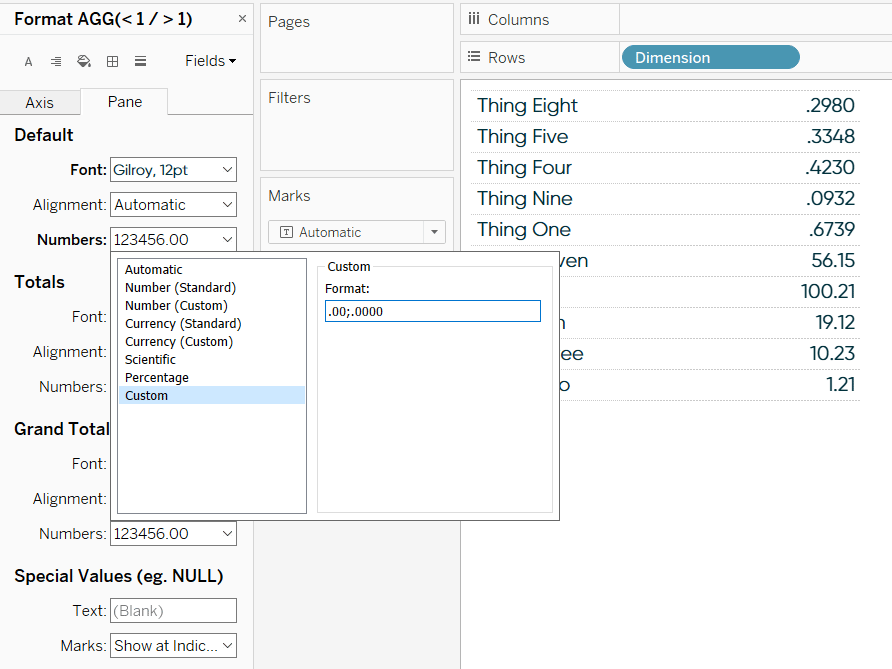Take a closer look at the table in the last image – values less than one display four decimal places, and values greater than one display just two! Again, we’re using this as an alternative solution to rounding decimal places, but you can create any second number formatting for your exception (the measure being multiplied by negative one). This could be different decimal places, a dollar sign, a percent sign, etc.

But wait – there’s more!

You can unlock a third number format by typing a second semicolon in this text box for custom number formatting. This is the format that will be displayed for values that equal zero. Let’s say that we don’t want to focus on the dimension members in our view that have already reached their goal for the year. Instead, I will add a star alt-code character (★) to communicate that we no longer need to worry about those items.

### Receive More Free Tips & Tricks Like This Every Week

Let’s stay in touch:First, I have to add a line of logic to my calculated field that converted some of our values to negative to also convert some of our values to zero. We’ll say our goal for the year is 50, so any dimension member that has reached that threshold will be converted to zero. The formula is:

IF SUM([Measure]) < 1 THEN -SUM([Measure])
ELSEIF SUM([Measure]) > 50 THEN 0
ELSE SUM([Measure])
END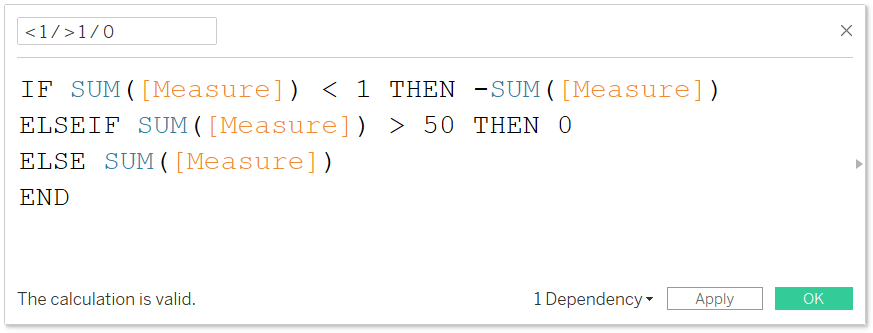In addition to displaying four decimal places for values less than one and two decimal places for values greater than one, this formula will now convert the values that are past our goal of 50 to zeros. Now if I go type a second semicolon into the custom formatting for our calculated measure, whatever number format I type next will be applied to the values that are above goal.

Here’s how the view looks when typing a star alt-code character as the third number formatting.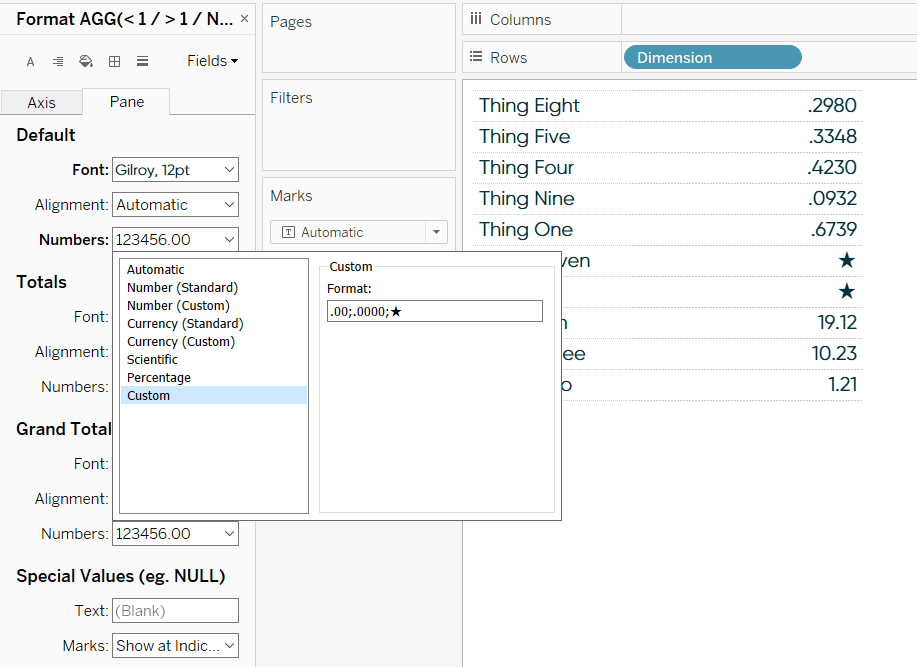If you would still like to display the actual value for any of these dimension members on the table, you can simply add the original measure to the Tooltip Marks Card. In other words, you can display a star to let the end user know they don’t need to worry about that item, but if they want to be reminded of the actual performance, they’ll see it when they hover over the marks.

The only caveat with this trick is that you can’t have negative values or zeros because those actual negatives and zeros would get this new custom format applied to them.

– Ryan

## Become a memberHow to Flexibly Format Decimal Places in Tableau

## Related Content

### 3 Ways to Use Alt Code Characters (▲,★,■) to Enhance Your Tableau Dashboards

ASCII, or American Standard Code for Information Interchange, is the standard when it comes to computers converting numbers into readable…

### How to Dynamically Format Numbers in TableauRyan Sleeper

3 ways to take command of Tableau number formatting Learn how to (1) easily format positive and negative changes with…

### 3 Ways to Conditionally Format Numbers in Tableau

There are many needs to conditionally format numbers in Tableau. I’ve previously covered how to dynamically change the prefix and…